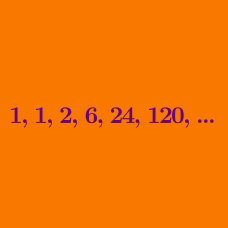Number Theory

# Factorials: Level 2 Challenges

Which of these numbers is a perfect square?

What is the product of all positive odd integers less than $10000$?

$\large 1 \cdot 1! + 2\cdot 2! + 3\cdot 3! + \cdots + 25\cdot 25! = \, ?$

Which is greater?

$300! \text{ or } 100^{300} ?$

Given that $13!= 13 \times 12 \times 11 \times \cdot \cdot \cdot \times 2 \times 1 = 6227020800$, it can be seen that $13!$ contains two trailing zeroes.

How many trailing zeroes does $1000!$ contain?

×